x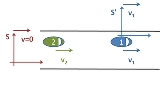Frame of referenceEncyclopedia
A frame of reference in physics
Physics
Physics is a natural science that involves the study of matter and its motion through spacetime, along with related concepts such as energy and force. More broadly, it is the general analysis of nature, conducted in order to understand how the universe behaves.Physics is one of the oldest academic...

, may refer to a coordinate system
Coordinate system
In geometry, a coordinate system is a system which uses one or more numbers, or coordinates, to uniquely determine the position of a point or other geometric element. The order of the coordinates is significant and they are sometimes identified by their position in an ordered tuple and sometimes by...

or set of axes
Cartesian coordinate system
A Cartesian coordinate system specifies each point uniquely in a plane by a pair of numerical coordinates, which are the signed distances from the point to two fixed perpendicular directed lines, measured in the same unit of length...

within which to measure the position, orientation
Orientation (geometry)
In geometry the orientation, angular position, or attitude of an object such as a line, plane or rigid body is part of the description of how it is placed in the space it is in....

, and other properties of objects in it, or it may refer to an observational reference frame tied to the state of motion of an observer
Observer (special relativity)
The term observer in special relativity refers most commonly to an inertial reference frame. Less often it may refer to an arbitrary non-inertial reference frame; in particular, a Rindler frame is sometimes called an "accelerating observer". In such cases an inertial reference frame may be called...

.
It may also refer to both an observational reference frame and an attached coordinate system as a unit.

## Different aspects of "frame of reference"

The need to distinguish between the various meanings of "frame of reference" has led to a variety of terms. For example, sometimes the type of coordinate system is attached as a modifier, as in Cartesian frame of reference. Sometimes the state of motion is emphasized, as in rotating frame of reference
Rotating reference frame
A rotating frame of reference is a special case of a non-inertial reference frame that is rotating relative to an inertial reference frame. An everyday example of a rotating reference frame is the surface of the Earth. A rotating frame of reference is a special case of a non-inertial reference...

. Sometimes the way it transforms to frames considered as related is emphasized as in Galilean frame of reference. Sometimes frames are distinguished by the scale of their observations, as in macroscopic and microscopic frames of reference.

In this article the term observational frame of reference is used when emphasis is upon the state of motion rather than upon the coordinate choice or the character of the observations or observational apparatus. In this sense, an observational frame of reference allows study of the effect of motion upon an entire family of coordinate systems that could be attached to this frame. On the other hand, a coordinate system may be employed for many purposes where the state of motion is not the primary concern. For example, a coordinate system may be adopted to take advantage of the symmetry of a system. In a still broader perspective, of course, the formulation of many problems in physics employs generalized coordinates
Generalized coordinates
In the study of multibody systems, generalized coordinates are a set of coordinates used to describe the configuration of a system relative to some reference configuration....

, normal modes or eigenvectors, which are only indirectly related to space and time. It seems useful to divorce the various aspects of a reference frame for the discussion below. We therefore take observational frames of reference, coordinate systems, and observational equipment as independent concepts, separated as below:
• An observational frame (such as an inertial frame or non-inertial frame of reference) is a physical concept related to state of motion.

• A coordinate system is a mathematical concept, amounting to a choice of language used to describe observations. Consequently, an observer in an observational frame of reference can choose to employ any coordinate system (Cartesian, polar, curvilinear, generalized, …) to describe observations made from that frame of reference. A change in the choice of this coordinate system does not change an observer's state of motion, and so does not entail a change in the observer's observational frame of reference. This viewpoint can be found elsewhere as well. Which is not to dispute that some coordinate systems may be a better choice for some observations than are others.

• Choice of what to measure and with what observational apparatus is a matter separate from the observer's state of motion and choice of coordinate system.

Here is a quotation applicable to moving observational frames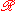and various associated Euclidean three-space coordinate systems [R, R' , etc.]:
and this on the utility of separating the notions ofand [R, R' , etc.]:

and this, also on the distinction betweenand [R, R' , etc.]:
and from J. D. Norton:
The discussion is taken beyond simple space-time coordinate systems by Brading and Castellani. Extension to coordinate systems using generalized coordinates underlies the Hamiltonian
Hamilton's principle
In physics, Hamilton's principle is William Rowan Hamilton's formulation of the principle of stationary action...

and Lagrangian
Lagrangian
The Lagrangian, L, of a dynamical system is a function that summarizes the dynamics of the system. It is named after Joseph Louis Lagrange. The concept of a Lagrangian was originally introduced in a reformulation of classical mechanics by Irish mathematician William Rowan Hamilton known as...

formulations of quantum field theory
Quantum field theory
Quantum field theory provides a theoretical framework for constructing quantum mechanical models of systems classically parametrized by an infinite number of dynamical degrees of freedom, that is, fields and many-body systems. It is the natural and quantitative language of particle physics and...

, classical relativistic mechanics
Classical mechanics
In physics, classical mechanics is one of the two major sub-fields of mechanics, which is concerned with the set of physical laws describing the motion of bodies under the action of a system of forces...

, and quantum gravity
Quantum gravity
Quantum gravity is the field of theoretical physics which attempts to develop scientific models that unify quantum mechanics with general relativity...

.

### Coordinate systems

Although the term "coordinate system" is often used (particularly by physicists) in a nontechnical sense, the term "coordinate system" does have a precise meaning in mathematics, and sometimes that is what the physicist means as well.

A coordinate system in mathematics is a facet of geometry
Geometry
Geometry arose as the field of knowledge dealing with spatial relationships. Geometry was one of the two fields of pre-modern mathematics, the other being the study of numbers ....

or of algebra
Algebra
Algebra is the branch of mathematics concerning the study of the rules of operations and relations, and the constructions and concepts arising from them, including terms, polynomials, equations and algebraic structures...

, in particular, a property of manifold
Manifold
In mathematics , a manifold is a topological space that on a small enough scale resembles the Euclidean space of a specific dimension, called the dimension of the manifold....

s (for example, in physics, configuration space
Configuration space
- Configuration space in physics :In classical mechanics, the configuration space is the space of possible positions that a physical system may attain, possibly subject to external constraints...

s or phase space
Phase space
In mathematics and physics, a phase space, introduced by Willard Gibbs in 1901, is a space in which all possible states of a system are represented, with each possible state of the system corresponding to one unique point in the phase space...

s). The coordinates
Cartesian coordinate system
A Cartesian coordinate system specifies each point uniquely in a plane by a pair of numerical coordinates, which are the signed distances from the point to two fixed perpendicular directed lines, measured in the same unit of length...

of a point r in an n-dimensional space are simply an ordered set of n numbers: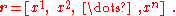In a general Banach space
Banach space
In mathematics, Banach spaces is the name for complete normed vector spaces, one of the central objects of study in functional analysis. A complete normed vector space is a vector space V with a norm ||·|| such that every Cauchy sequence in V has a limit in V In mathematics, Banach spaces is the...

, these numbers could be (for example) coefficients in a functional expansion like a Fourier series
Fourier series
In mathematics, a Fourier series decomposes periodic functions or periodic signals into the sum of a set of simple oscillating functions, namely sines and cosines...

. In a physical problem, they could be spacetime
Spacetime
In physics, spacetime is any mathematical model that combines space and time into a single continuum. Spacetime is usually interpreted with space as being three-dimensional and time playing the role of a fourth dimension that is of a different sort from the spatial dimensions...

coordinates or normal mode
Normal mode
A normal mode of an oscillating system is a pattern of motion in which all parts of the system move sinusoidally with the same frequency and with a fixed phase relation. The frequencies of the normal modes of a system are known as its natural frequencies or resonant frequencies...

amplitudes. In a robot design
Robotics
Robotics is the branch of technology that deals with the design, construction, operation, structural disposition, manufacture and application of robots...

, they could be angles of relative rotations, linear displacements, or deformations of joints
A mechanical linkage is an assembly of bodies connected together to manage forces and movement. The movement of a body, or link, is studied using geometry so the link is considered to be rigid. The connections between links are modeled as providing ideal movement, pure rotation or sliding for...

. Here we will suppose these coordinates can be related to a Cartesian coordinate system by a set of functions: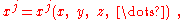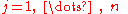where x, y, z, etc. are the n Cartesian coordinates of the point. Given these functions, coordinate surfaces are defined by the relations: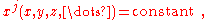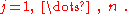The intersection of these surfaces define coordinate lines. At any selected point, tangents to the intersecting coordinate lines at that point define a set of basis vectors {e1, e2, …, en} at that point. That is: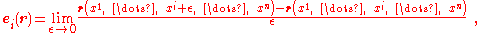which can be normalized to be of unit length. For more detail see curvilinear coordinates.

Coordinate surfaces, coordinate lines, and basis vectors
Basis (linear algebra)
In linear algebra, a basis is a set of linearly independent vectors that, in a linear combination, can represent every vector in a given vector space or free module, or, more simply put, which define a "coordinate system"...

are components of a coordinate system. If the basis vectors are orthogonal at every point, the coordinate system is an orthogonal coordinate system
Orthogonal coordinates
In mathematics, orthogonal coordinates are defined as a set of d coordinates q = in which the coordinate surfaces all meet at right angles . A coordinate surface for a particular coordinate qk is the curve, surface, or hypersurface on which qk is a constant...

.

An important aspect of a coordinate system is its metric
Metric (mathematics)
In mathematics, a metric or distance function is a function which defines a distance between elements of a set. A set with a metric is called a metric space. A metric induces a topology on a set but not all topologies can be generated by a metric...

gik, which determines the arc length
Arc length
Determining the length of an irregular arc segment is also called rectification of a curve. Historically, many methods were used for specific curves...

ds in the coordinate system in terms of its coordinates: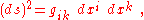where repeated indices are summed over.

As is apparent from these remarks, a coordinate system is a mathematical construct
Model theory
In mathematics, model theory is the study of mathematical structures using tools from mathematical logic....

, part of an axiomatic system
Axiomatic system
In mathematics, an axiomatic system is any set of axioms from which some or all axioms can be used in conjunction to logically derive theorems. A mathematical theory consists of an axiomatic system and all its derived theorems...

. There is no necessary connection between coordinate systems and physical motion (or any other aspect of reality). However, coordinate systems can include time as a coordinate, and can be used to describe motion. Thus, Lorentz transformation
Lorentz transformation
In physics, the Lorentz transformation or Lorentz-Fitzgerald transformation describes how, according to the theory of special relativity, two observers' varying measurements of space and time can be converted into each other's frames of reference. It is named after the Dutch physicist Hendrik...

s and Galilean transformation
Galilean transformation
The Galilean transformation is used to transform between the coordinates of two reference frames which differ only by constant relative motion within the constructs of Newtonian physics. This is the passive transformation point of view...

s may be viewed as coordinate transformations.

### Observational frames of reference

An observational frame of reference, often referred to as a physical frame of reference, a frame of reference, or simply a frame, is a physical concept related to an observer
Observer (special relativity)
The term observer in special relativity refers most commonly to an inertial reference frame. Less often it may refer to an arbitrary non-inertial reference frame; in particular, a Rindler frame is sometimes called an "accelerating observer". In such cases an inertial reference frame may be called...

and the observer's state of motion. Here we adopt the view expressed by Kumar and Barve: an observational frame of reference is characterized only by its state of motion. However, there is lack of unanimity on this point. In special relativity, the distinction is sometimes made between an observer and a frame. According to this view, a frame is an observer plus a coordinate lattice constructed to be an orthonormal right-handed set of spacelike vectors perpendicular to a timelike vector. See Doran. This restricted view is not used here, and is not universally adopted even in discussions of relativity. In general relativity
General relativity
General relativity or the general theory of relativity is the geometric theory of gravitation published by Albert Einstein in 1916. It is the current description of gravitation in modern physics...

the use of general coordinate systems is common (see, for example, the Schwarzschild
Karl Schwarzschild
Karl Schwarzschild was a German physicist. He is also the father of astrophysicist Martin Schwarzschild.He is best known for providing the first exact solution to the Einstein field equations of general relativity, for the limited case of a single spherical non-rotating mass, which he accomplished...

solution for the gravitational field outside an isolated sphere).

There are two types of observational reference frame: inertial
Inertial frame of reference
In physics, an inertial frame of reference is a frame of reference that describes time homogeneously and space homogeneously, isotropically, and in a time-independent manner.All inertial frames are in a state of constant, rectilinear motion with respect to one another; they are not...

and non-inertial
Non-inertial reference frame
A non-inertial reference frame is a frame of reference that is under acceleration. The laws of physics in such a frame do not take on their most simple form, as required by the theory of special relativity...

. An inertial frame of reference is defined as one in which all laws of physics take on their simplest form. In special relativity
Special relativity
Special relativity is the physical theory of measurement in an inertial frame of reference proposed in 1905 by Albert Einstein in the paper "On the Electrodynamics of Moving Bodies".It generalizes Galileo's...

these frames are related by Lorentz transformation
Lorentz transformation
In physics, the Lorentz transformation or Lorentz-Fitzgerald transformation describes how, according to the theory of special relativity, two observers' varying measurements of space and time can be converted into each other's frames of reference. It is named after the Dutch physicist Hendrik...

s. In Newtonian mechanics, a more restricted definition requires only that Newton's first law holds true; that is, a Newtonian inertial frame is one in which a free particle
Free particle
In physics, a free particle is a particle that, in some sense, is not bound. In classical physics, this means the particle is present in a "field-free" space.-Classical Free Particle:The classical free particle is characterized simply by a fixed velocity...

travels in a straight line at constant speed
Speed
In kinematics, the speed of an object is the magnitude of its velocity ; it is thus a scalar quantity. The average speed of an object in an interval of time is the distance traveled by the object divided by the duration of the interval; the instantaneous speed is the limit of the average speed as...

, or is at rest. These frames are related by Galilean transformation
Galilean transformation
The Galilean transformation is used to transform between the coordinates of two reference frames which differ only by constant relative motion within the constructs of Newtonian physics. This is the passive transformation point of view...

s. These relativistic and Newtonian transformations are expressed in spaces of general dimension in terms of representations
Representation theory
Representation theory is a branch of mathematics that studies abstract algebraic structures by representing their elements as linear transformations of vector spaces, and studiesmodules over these abstract algebraic structures...

of the Poincaré group
Representation theory of the Poincaré group
In mathematics, the representation theory of the Poincaré group is an example of the representation theory of a Lie group that is neither a compact group nor a semisimple group. It is fundamental in theoretical physics....

and of the Galilean group
Representation theory of the Galilean group
In nonrelativistic quantum mechanics, an account can be given of the existence of mass and spin as follows:The spacetime symmetry group of nonrelativistic quantum mechanics is the Galilean group...

.

In contrast to the inertial frame, a non-inertial frame of reference is one in which fictitious force
Fictitious force
A fictitious force, also called a pseudo force, d'Alembert force or inertial force, is an apparent force that acts on all masses in a non-inertial frame of reference, such as a rotating reference frame....

s must be invoked to explain observations. An example is an observational frame of reference centered at a point on the Earth's surface. This frame of reference orbits around the center of the Earth, which introduces a fictitious force known as the Coriolis force (among others).

### Measurement apparatus

A further aspect of a frame of reference is the role of the measurement apparatus
Metrology
Metrology is the science of measurement. Metrology includes all theoretical and practical aspects of measurement. The word comes from Greek μέτρον , "measure" + "λόγος" , amongst others meaning "speech, oration, discourse, quote, study, calculation, reason"...

(for example, clocks and rods) attached to the frame (see Norton quote above). This question is not addressed in this article, and is of particular interest in quantum mechanics
Measurement in quantum mechanics
The framework of quantum mechanics requires a careful definition of measurement. The issue of measurement lies at the heart of the problem of the interpretation of quantum mechanics, for which there is currently no consensus....

, where the relation between observer and measurement is still under discussion (see measurement problem
Measurement problem
The measurement problem in quantum mechanics is the unresolved problem of how wavefunction collapse occurs. The inability to observe this process directly has given rise to different interpretations of quantum mechanics, and poses a key set of questions that each interpretation must answer...

).

In physics experiments, the frame of reference in which the laboratory measurement devices are at rest is usually referred to as the laboratory frame or simply "lab frame." An example would be the frame in which the detectors for a particle accelerator are at rest. The lab frame in some experiments is an inertial frame, but it is not required to be (for example the laboratory in the surface of the Earth in many physics experiments is not inertial). In particle physics experiments, it is often useful to transform energies and momenta of particles from the lab frame where they are measured, to the center of momentum frame
Center of momentum frame
A center-of-momentum frame of a system is any inertial frame in which the center of mass is at rest . Note that the center of momentum of a system is not a location, but rather defines a particular inertial frame...

"COM frame" in which calculations are sometimes simplified, since potentially all kinetic energy still present in the COM frame may be used for making new particles.

In this connection it may be noted that the clocks and rods often used to describe observers' measurement equipment in thought, in practice are replaced by a much more complicated and indirect metrology
Metrology
Metrology is the science of measurement. Metrology includes all theoretical and practical aspects of measurement. The word comes from Greek μέτρον , "measure" + "λόγος" , amongst others meaning "speech, oration, discourse, quote, study, calculation, reason"...

that is connected to the nature of the vacuum
Vacuum
In everyday usage, vacuum is a volume of space that is essentially empty of matter, such that its gaseous pressure is much less than atmospheric pressure. The word comes from the Latin term for "empty". A perfect vacuum would be one with no particles in it at all, which is impossible to achieve in...

, and uses atomic clocks that operate according to the standard model
Standard Model
The Standard Model of particle physics is a theory concerning the electromagnetic, weak, and strong nuclear interactions, which mediate the dynamics of the known subatomic particles. Developed throughout the mid to late 20th century, the current formulation was finalized in the mid 1970s upon...

and that must be corrected for gravitational time dilation
Gravitational time dilation
Gravitational time dilation is the effect of time passing at different rates in regions of different gravitational potential; the lower the gravitational potential, the more slowly time passes...

. (See second
Second
The second is a unit of measurement of time, and is the International System of Units base unit of time. It may be measured using a clock....

, meter and kilogram
Kilogram
The kilogram or kilogramme , also known as the kilo, is the base unit of mass in the International System of Units and is defined as being equal to the mass of the International Prototype Kilogram , which is almost exactly equal to the mass of one liter of water...

).

In fact, Einstein felt that clocks and rods were merely expedient measuring devices and they should be replaced by more fundamental entities based upon, for example, atoms and molecules.

### Simple example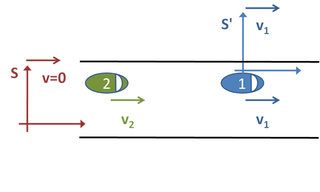Consider a situation common in everyday life. Two cars travel along a road, both moving at a constant velocity. See Figure 1. At some particular moment, they are separated by 200 metres. The car in front is travelling at 22 metres per second and the car behind is travelling at 30 metres per second. If we want to find out how long it will take the second car to catch up with the first, there are three obvious "frames of reference" that we could choose.

First, we could observe the two cars from the side of the road. We define our "frame of reference" S as follows. We stand on the side of the road and start a stop-clock at the exact moment that the second car passes us, which happens to be when they are a distance d = 200 m apart. Since neither of the cars is accelerating, we can determine their positions by the following formulas, where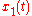is the position in meters of car one after time t seconds and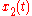is the position of car two after time t.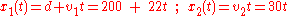Notice that these formulas predict at t = 0 s the first car is 200 m down the road and the second car is right beside us, as expected. We want to find the time at which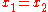. Therefore we setand solve for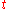, that is: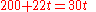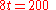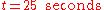Alternatively, we could choose a frame of reference S' situated in the first car. In this case, the first car is stationary and the second car is approaching from behind at a speed of v2 − v1 = 8 m / s. In order to catch up to the first car, it will take a time of d /( v2 − v1) = 200 / 8 s, that is, 25 seconds, as before. Note how much easier the problem becomes by choosing a suitable frame of reference. The third possible frame of reference would be attached to the second car. That example resembles the case just discussed, except the second car is stationary and the first car moves backward towards it at 8 m / s.

It would have been possible to choose a rotating, accelerating frame of reference, moving in a complicated manner, but this would have served to complicate the problem unnecessarily. It is also necessary to note that one is able to convert measurements made in one coordinate system to another. For example, suppose that your watch is running five minutes fast compared to the local standard time. If you know that this is the case, when somebody asks you what time it is, you are able to deduct five minutes from the time displayed on your watch in order to obtain the correct time. The measurements that an observer makes about a system depend therefore on the observer's frame of reference (you might say that the bus arrived at 5 past three, when in fact it arrived at three).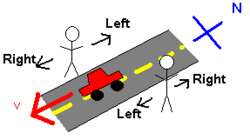For a simple example involving only the orientation of two observers, consider two people standing, facing each other on either side of a north-south street. See Figure 2. A car drives past them heading south. For the person facing east, the car was moving toward the right. However, for the person facing west, the car was moving toward the left. This discrepancy is because the two people used two different frames of reference from which to investigate this system.

For a more complex example involving observers in relative motion, consider Alfred, who is standing on the side of a road watching a car drive past him from left to right. In his frame of reference, Alfred defines the spot where he is standing as the origin, the road as the x-axis and the direction in front of him as the positive y-axis. To him, the car moves along the x axis with some velocity
Velocity
In physics, velocity is speed in a given direction. Speed describes only how fast an object is moving, whereas velocity gives both the speed and direction of the object's motion. To have a constant velocity, an object must have a constant speed and motion in a constant direction. Constant ...

v in the positive x-direction. Alfred's frame of reference is considered an inertial frame of reference
Inertial frame of reference
In physics, an inertial frame of reference is a frame of reference that describes time homogeneously and space homogeneously, isotropically, and in a time-independent manner.All inertial frames are in a state of constant, rectilinear motion with respect to one another; they are not...

because he is not accelerating (ignoring effects such as Earth's rotation and gravity).

Now consider Betsy, the person driving the car. Betsy, in choosing her frame of reference, defines her location as the origin, the direction to her right as the positive x-axis, and the direction in front of her as the positive y-axis. In this frame of reference, it is Betsy who is stationary and the world around her that is moving - for instance, as she drives past Alfred, she observes him moving with velocity v in the negative y-direction. If she is driving north, then north is the positive y-direction; if she turns east, east becomes the positive y-direction.

Finally, as an example of non-inertial observers, assume Candace is accelerating her car. As she passes by him, Alfred measures her acceleration
Acceleration
In physics, acceleration is the rate of change of velocity with time. In one dimension, acceleration is the rate at which something speeds up or slows down. However, since velocity is a vector, acceleration describes the rate of change of both the magnitude and the direction of velocity. ...

and finds it to be a in the negative x-direction. Assuming Candace's acceleration is constant, what acceleration does Betsy measure? If Betsy's velocity v is constant, she is in an inertial frame of reference, and she will find the acceleration to be the same as Alfred - in her frame of reference, a in the negative y-direction. However, if she is accelerating at rate A in the negative y-direction (in other words, slowing down), she will find Candace's acceleration to be a' = a - A in the negative y-direction - a smaller value than Alfred has measured. Similarly, if she is accelerating at rate A in the positive y-direction (speeding up), she will observe Candace's acceleration as a' = a + A in the negative y-direction - a larger value than Alfred's measurement.

Frames of reference are especially important in special relativity
Special relativity
Special relativity is the physical theory of measurement in an inertial frame of reference proposed in 1905 by Albert Einstein in the paper "On the Electrodynamics of Moving Bodies".It generalizes Galileo's...

, because when a frame of reference is moving at some significant fraction of the speed of light, then the flow of time in that frame does not necessarily apply in another frame. The speed of light is considered to be the only true constant between moving frames of reference.

### Remarks

It is important to note some assumptions made above about the various inertial frames of reference. Newton, for instance, employed universal time, as explained by the following example. Suppose that you own two clocks, which both tick at exactly the same rate. You synchronize them so that they both display exactly the same time. The two clocks are now separated and one clock is on a fast moving train, traveling at constant velocity towards the other. According to Newton, these two clocks will still tick at the same rate and will both show the same time. Newton says that the rate of time as measured in one frame of reference should be the same as the rate of time in another. That is, there exists a "universal" time and all other times in all other frames of reference will run at the same rate as this universal time irrespective of their position and velocity. This concept of time and simultaneity was later generalized by Einstein in his special theory of relativity (1905) where he developed transformations between inertial frames of reference based upon the universal nature of physical laws and their economy of expression (Lorentz transformations).

It is also important to note that the definition of inertial reference frame can be extended beyond three dimensional Euclidean space. Newton's assumed a Euclidean space, but general relativity
General relativity
General relativity or the general theory of relativity is the geometric theory of gravitation published by Albert Einstein in 1916. It is the current description of gravitation in modern physics...

uses a more general geometry. As an example of why this is important, let us consider the geometry
Geometry
Geometry arose as the field of knowledge dealing with spatial relationships. Geometry was one of the two fields of pre-modern mathematics, the other being the study of numbers ....

of an ellipsoid. In this geometry, a "free" particle is defined as one at rest or traveling at constant speed on a geodesic
Geodesic
In mathematics, a geodesic is a generalization of the notion of a "straight line" to "curved spaces". In the presence of a Riemannian metric, geodesics are defined to be the shortest path between points in the space...

path. Two free particles may begin at the same point on the surface, traveling with the same constant speed in different directions. After a length of time, the two particles collide at the opposite side of the ellipsoid. Both "free" particles traveled with a constant speed, satisfying the definition that no forces were acting. No acceleration occurred and so Newton's first law held true. This means that the particles were in inertial frames of reference. Since no forces were acting, it was the geometry of the situation which caused the two particles to meet each other again. In a similar way, it is now believed that we exist in a four dimensional geometry known as spacetime
Spacetime
In physics, spacetime is any mathematical model that combines space and time into a single continuum. Spacetime is usually interpreted with space as being three-dimensional and time playing the role of a fourth dimension that is of a different sort from the spatial dimensions...

. It is believed that the curvature of this 4D space is responsible for the way in which two bodies with mass are drawn together even if no forces are acting. This curvature of spacetime replaces the force known as gravity in Newtonian mechanics and special relativity.

## Non-inertial frames

Here the relation between inertial and non-inertial observational frames of reference is considered. The basic difference between these frames is the need in non-inertial frames for fictitious forces, as described below.

An accelerated frame of reference is often delineated as being the "primed" frame, and all variables that are dependent on that frame are notated with primes, e.g. x' , y' , a' .

The vector from the origin of an inertial reference frame to the origin of an accelerated reference frame is commonly notated as R. Given a point of interest that exists in both frames, the vector from the inertial origin to the point is called r, and the vector from the accelerated origin to the point is called r'.
From the geometry of the situation, we get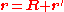Taking the first and second derivatives of this, we obtain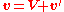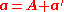where V and A are the velocity and acceleration of the accelerated system with respect to the inertial system and v and a are the velocity and acceleration of the point of interest with respect to the inertial frame.

These equations allow transformations between the two coordinate systems; for example, we can now write Newton's second law as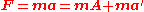When there is accelerated motion due to a force being exerted there is manifestation of inertia. If an electric car designed to recharge its battery system when decelerating is switched to braking, the batteries are recharged, illustrating the physical strength of manifestation of inertia. However, the manifestation of inertia does not prevent acceleration (or deceleration), for manifestation of inertia occurs in response to change in velocity due to a force. Seen from the perspective of a rotating frame of reference the manifestation of inertia appears to exert a force (either in centrifugal direction, or in a direction orthogonal to an object's motion, the Coriolis effect
Coriolis effect
In physics, the Coriolis effect is a deflection of moving objects when they are viewed in a rotating reference frame. In a reference frame with clockwise rotation, the deflection is to the left of the motion of the object; in one with counter-clockwise rotation, the deflection is to the right...

).

A common sort of accelerated reference frame is a frame that is both rotating and translating (an example is a frame of reference attached to a CD which is playing while the player is carried). This arrangement leads to the equation (see Fictitious force
Fictitious force
A fictitious force, also called a pseudo force, d'Alembert force or inertial force, is an apparent force that acts on all masses in a non-inertial frame of reference, such as a rotating reference frame....

for a derivation):or, to solve for the acceleration in the accelerated frame,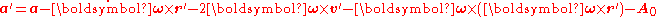Multiplying through by the mass m gives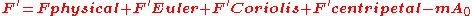where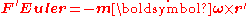(Euler force
Euler force
In classical mechanics, the Euler acceleration , also known as azimuthal acceleration or transverse acceleration is an acceleration that appears when a non-uniformly rotating reference frame is used for analysis of motion and there is variation in the angular velocity of the reference frame's axes...

)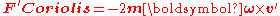(Coriolis force)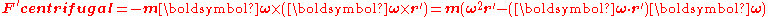(centrifugal force)

## Particular frames of reference in common use

• International Terrestrial Reference Frame
• International Celestial Reference Frame
International Celestial Reference Frame
The International Celestial Reference Frame is a quasi-inertial reference frame centered at the barycenter of the Solar System, defined by the measured positions of 212 extragalactic sources . Although relativity implies that there is no true inertial frame, the extragalactic sources used to...

• In fluid mechanics, Lagrangian and Eulerian specification of the flow field

### Other frames

• Cognitive psychology
Cognitive psychology
Cognitive psychology is a subdiscipline of psychology exploring internal mental processes.It is the study of how people perceive, remember, think, speak, and solve problems.Cognitive psychology differs from previous psychological approaches in two key ways....

• Frame fields in general relativity
Frame fields in general relativity
In general relativity, a frame field is a set of four orthonormal vector fields, one timelike and three spacelike, defined on a Lorentzian manifold that is physically interpreted as a model of spacetime...

• Linguistic frame of reference
Linguistic frame of reference
Linguistic frame of reference is a frame of reference as it is expressed in a language.A frame of reference is a coordinate system used to identify location of an object....

• Moving frame in Mathematics
Moving frame
In mathematics, a moving frame is a flexible generalization of the notion of an ordered basis of a vector space often used to study the extrinsic differential geometry of smooth manifolds embedded in a homogeneous space.-Introduction:...

• Analytical mechanics
Analytical mechanics
Analytical mechanics is a term used for a refined, mathematical form of classical mechanics, constructed from the 18th century onwards as a formulation of the subject as founded by Isaac Newton. Often the term vectorial mechanics is applied to the form based on Newton's work, to contrast it with...

• Applied mechanics
Applied mechanics
Applied mechanics is a branch of the physical sciences and the practical application of mechanics. Applied mechanics examines the response of bodies or systems of bodies to external forces...

• Cartesian coordinate system
Cartesian coordinate system
A Cartesian coordinate system specifies each point uniquely in a plane by a pair of numerical coordinates, which are the signed distances from the point to two fixed perpendicular directed lines, measured in the same unit of length...

• Centrifugal force
Centrifugal force
Centrifugal force can generally be any force directed outward relative to some origin. More particularly, in classical mechanics, the centrifugal force is an outward force which arises when describing the motion of objects in a rotating reference frame...

• Centripetal force
Centripetal force
Centripetal force is a force that makes a body follow a curved path: it is always directed orthogonal to the velocity of the body, toward the instantaneous center of curvature of the path. The mathematical description was derived in 1659 by Dutch physicist Christiaan Huygens...

• Classical mechanics
Classical mechanics
In physics, classical mechanics is one of the two major sub-fields of mechanics, which is concerned with the set of physical laws describing the motion of bodies under the action of a system of forces...

• Coriolis force
• Curvilinear coordinates
Curvilinear coordinates
Curvilinear coordinates are a coordinate system for Euclidean space in which the coordinate lines may be curved. These coordinates may be derived from a set of Cartesian coordinates by using a transformation that is locally invertible at each point. This means that one can convert a point given...

• Cylindrical coordinate system
Cylindrical coordinate system
A cylindrical coordinate system is a three-dimensional coordinate systemthat specifies point positions by the distance from a chosen reference axis, the direction from the axis relative to a chosen reference direction, and the distance from a chosen reference plane perpendicular to the axis...

• Dynamics (physics)
• Fictitious force
Fictitious force
A fictitious force, also called a pseudo force, d'Alembert force or inertial force, is an apparent force that acts on all masses in a non-inertial frame of reference, such as a rotating reference frame....

• Frenet-Serret formulas
Frenet-Serret formulas
In vector calculus, the Frenet–Serret formulas describe the kinematic properties of a particle which moves along a continuous, differentiable curve in three-dimensional Euclidean space R3...

• Galilean invariance
Galilean invariance
Galilean invariance or Galilean relativity is a principle of relativity which states that the fundamental laws of physics are the same in all inertial frames...

• General relativity
General relativity
General relativity or the general theory of relativity is the geometric theory of gravitation published by Albert Einstein in 1916. It is the current description of gravitation in modern physics...

• Generalized coordinates
Generalized coordinates
In the study of multibody systems, generalized coordinates are a set of coordinates used to describe the configuration of a system relative to some reference configuration....

• Generalized forces
• Inertial frame of reference
Inertial frame of reference
In physics, an inertial frame of reference is a frame of reference that describes time homogeneously and space homogeneously, isotropically, and in a time-independent manner.All inertial frames are in a state of constant, rectilinear motion with respect to one another; they are not...

• Kinematics
Kinematics
Kinematics is the branch of classical mechanics that describes the motion of bodies and systems without consideration of the forces that cause the motion....

• Lorentz transformation
Lorentz transformation
In physics, the Lorentz transformation or Lorentz-Fitzgerald transformation describes how, according to the theory of special relativity, two observers' varying measurements of space and time can be converted into each other's frames of reference. It is named after the Dutch physicist Hendrik...

• Mach's principle
Mach's principle
In theoretical physics, particularly in discussions of gravitation theories, Mach's principle is the name given by Einstein to an imprecise hypothesis often credited to the physicist and philosopher Ernst Mach....

• Orthogonal coordinates
Orthogonal coordinates
In mathematics, orthogonal coordinates are defined as a set of d coordinates q = in which the coordinate surfaces all meet at right angles . A coordinate surface for a particular coordinate qk is the curve, surface, or hypersurface on which qk is a constant...

• Principle of relativity
Principle of relativity
In physics, the principle of relativity is the requirement that the equations describing the laws of physics have the same form in all admissible frames of reference....

• Special relativity
Special relativity
Special relativity is the physical theory of measurement in an inertial frame of reference proposed in 1905 by Albert Einstein in the paper "On the Electrodynamics of Moving Bodies".It generalizes Galileo's...

• Spherical coordinate system
Spherical coordinate system
In mathematics, a spherical coordinate system is a coordinate system for three-dimensional space where the position of a point is specified by three numbers: the radial distance of that point from a fixed origin, its inclination angle measured from a fixed zenith direction, and the azimuth angle of...

• Theory of relativity
Theory of relativity
The theory of relativity, or simply relativity, encompasses two theories of Albert Einstein: special relativity and general relativity. However, the word relativity is sometimes used in reference to Galilean invariance....

• Toroidal coordinates
Toroidal coordinates
Toroidal coordinates are a three-dimensional orthogonal coordinate system that results from rotating the two-dimensional bipolar coordinate system about the axis that separates its two foci. Thus, the two foci F_1 and F_2 in bipolar coordinates become a ring of radius a in the xy plane of the...